# 555 Timer Astable Multivibrator Circuit

Published  September 29, 2015   5
J
Author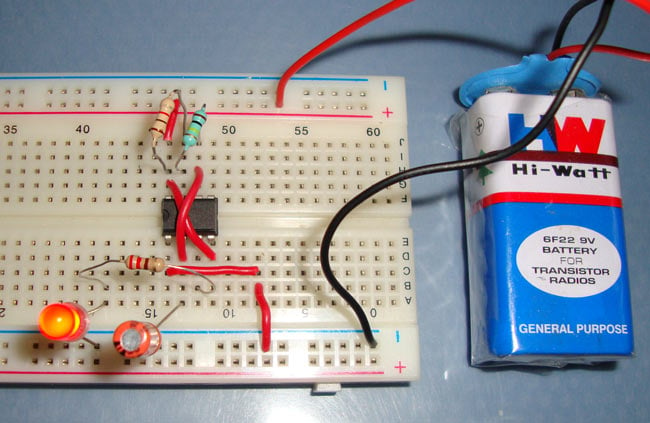Astable Multivibrator mode of 555 timer IC is also called Free running or self-triggering mode. Unlike Monostable Multivibrator mode it doesn’t have any stable state, it has two quasi stable state (HIGH and LOW). No external triggering is required in Astable mode, it automatically interchange its two states on a particular interval, hence generates a rectangular waveform. This time duration of HIGH and LOW output has been determined by the external resistors (R1 and R2) and a capacitor(C1). Astable mode works as a oscillator circuit, in which output oscillate at a particular frequency and generate pulses in rectangular wave form.

Using 555 timer IC, we can generate precise time duration of HIGH and LOW output, from micro seconds to hours, that’s why 555 is very popular and versatile IC. Before going through below, you should know about 555 timer IC and its PINs, here is the short description about its PINs.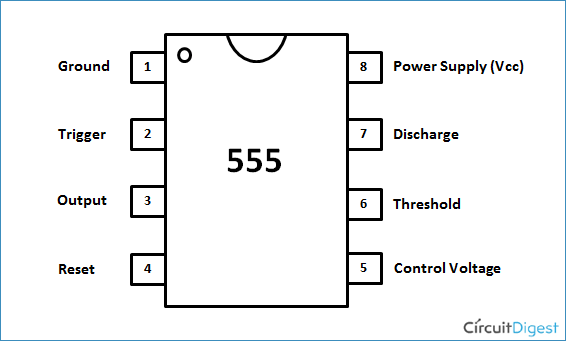Pin 1. Ground: This pin should be connected to ground.

Pin 2. TRIGGER:  Trigger pin is dragged from the negative input of comparator two. The Lower comparator output is connected to SET pin of flip-flop. A negative pulse (< Vcc/3) on this Pin sets the Flip flop and output goes High.

Pin 3. OUTPUT: This pin also has no special function. This is output pin where Load is connected. It can be used as source or sink and drive upto 200mA current.

Pin 4. Reset: There is a flip-flop in the timer chip. Reset pin is directly connected to MR (Master Reset) of the flip-flop. This is a active Low pin and normally connected to VCC for preventing accidental Reset.

Pin 5. Control Pin:  The control pin is connected from the negative input pin of comparator one. Output Pulse width can be controlled by applying voltage at this Pin, irrespective of RC network. Normally this pin is pulled down with a capacitor (0.01uF), to avoid unwanted noise interference with the working.

Pin 6. THRESHOLD: Threshold pin voltage determines when to reset the flip-flop in the timer. The threshold pin is drawn from positive input of upper comparator. If the control pin is open, then a voltage equal to or greater than VCC*(2/3) will reset the flip-flop. So the output goes low.

Pin 7. DISCHARGE: This pin is drawn from the open collector of transistor. Since the transistor (on which discharge pin got taken, Q1) got its base connected to Qbar. Whenever the output goes low or the flip-flop gets reset, the discharge pin is pulled to ground and capacitor discharges.

Pin 8. Power or VCC:  It is connected to positive voltage (+3.6v to +15v).

### Operation of Astable Multivibrator mode of 555 timer IC: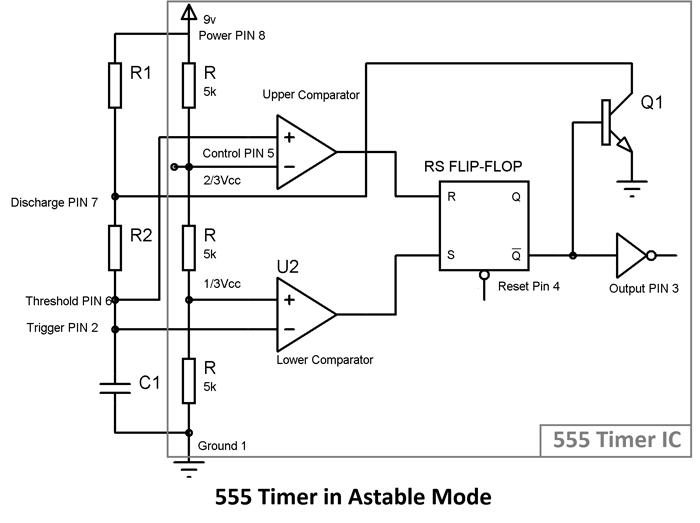• When initially power is turned ON, Trigger Pin voltage is below Vcc/3, that makes the lower comparator output HIGH and SETS the flip flop and output of the 555 chip is HIGH.
• This makes the transistor Q1 OFF, because Qbar, Q’=0 is directly applied to base of transistor. As the transistor is OFF, capacitor C1 starts charging and when it gets charged to a voltage above than Vcc/3, then Lower comparator output becomes LOW (Upper comparator is also at LOW) and Flip flop output remains the same as previous (555 output remains HIGH).
• Now when capacitor charging gets to voltage above than 2/3Vcc, then the voltage of non-inverting end (Threshold PIN 6) becomes higher than the inverting end of the comparator. This makes Upper comparator output HIGH and RESETs the Flip flop, output of 555 chip becomes LOW.
• As soon as the output of 555 get LOW means Q’=1, then transistor Q1 becomes ON and short the capacitor C1 to the Ground. So the capacitor C1 starts discharging to the ground through the Discharge PIN 7 and resistor R2.
• As capacitor voltage get down below the 2/3 Vcc, upper comparator output becomes LOW, now SR Flip flop remains in the previous state as both the comparators are LOW.
• While discharging, when capacitor voltage gets down below Vcc/3, this makes the Lower comparator output HIGH (upper comparator remain LOW) and Sets the flip flop again and 555 output becomes HIGH.
• Transistor Q1 becomes OFF and again capacitor C1 starts charging.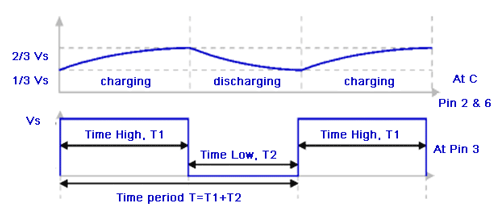This charging and discharging of capacitor continues and a rectangular oscillating output wave for is generated. While capacitor is getting charge the output of 555 is HIGH, and while capacitor is getting discharge output will be LOW. So this is called Astable mode because none of the state is stable and 555 automatically interchange its state from HIGH to LOW and LOW to HIGH, so it is called Free running Multivibrator.

Now the OUTPUT HIGH and OUTPUT LOW duration, is determined by the Resistors R1 & R2 and capacitor C1. This can be calculated using below formulas:

Time High (Seconds) T1 = 0.693 * (R1+R2) * C1

Time Low (Seconds) T2 = 0.693 * R2 * C1

Time Period T = Time High + Time Low = 0.693 * (R1+2*R2) * C1

Freqeuncy f = 1/Time Period = 1/ 0.693 * (R1+2*R2) * C1 = 1.44 / (R1+2*R2) * C1

Duty Cycle: Duty cycle is the ratio of time for which the output is HIGH to the total time.

Duty cycle %: (Time HIGH/ Total time) * 100 = (T1/T) * 100 = (R1+R2)/ (R1+2*R2) *100

You can also use this 555 Timer Astable Calculator to calculate above values.

Here is the practical demonstration of the Astable mode of 555 timer IC, where we have connected a LED to the output of the 555 IC. In this 555 astable multivibrator circuit, LED will switch ON and OFF automatically with a particular duration. ON time, OFF time, Frequency etc can be calculated using above formulas.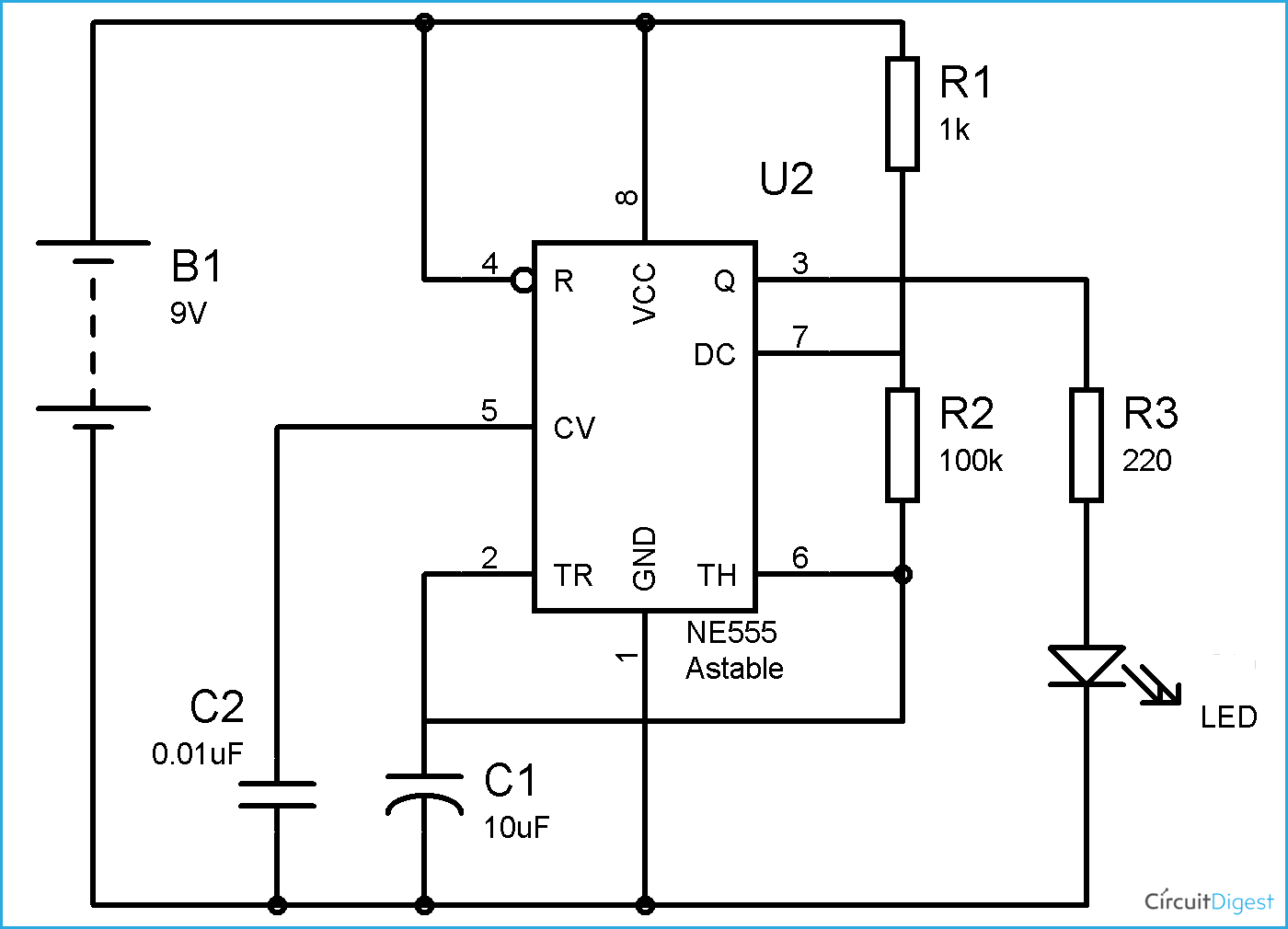Above figure shows the 555 timer astable multivibrator circuit diagram. You can find a lot of circuits and applications using astable mode in 555 timer circuits.

Video

Submitted by Vidyasagar Mandal on Sat, 04/01/2017 - 19:40

### How should be capacitor value

How should be capacitor value of 555 ic's 5 number pin

Submitted by GODWIN on Wed, 10/25/2017 - 19:38

### driving a motor with an astable multivibrator.

good afternoon
please i need a guide on how to switch between a positive and negative power supply at interval.
i want to drive a motor forward and reverse direction at intervals.

Submitted by ajay sharma on Wed, 11/15/2017 - 21:22

### GEC

It is more helpful to working on new project provided by any institutions or organization .it is huge amount of knowledge to help on new project and observe it experience is far better on theoretical knowledge.

Submitted by VIKRAM SINGH on Mon, 03/12/2018 - 16:15